Triangles - Olympiad Level MCQ, Class 10 Mathematics

# Triangles - Olympiad Level MCQ, Class 10 Mathematics

Test Description

## 25 Questions MCQ Test Olympiad Preparation for Class 10 | Triangles - Olympiad Level MCQ, Class 10 Mathematics

Triangles - Olympiad Level MCQ, Class 10 Mathematics for Class 10 2022 is part of Olympiad Preparation for Class 10 preparation. The Triangles - Olympiad Level MCQ, Class 10 Mathematics questions and answers have been prepared according to the Class 10 exam syllabus.The Triangles - Olympiad Level MCQ, Class 10 Mathematics MCQs are made for Class 10 2022 Exam. Find important definitions, questions, notes, meanings, examples, exercises, MCQs and online tests for Triangles - Olympiad Level MCQ, Class 10 Mathematics below.
 1 Crore+ students have signed up on EduRev. Have you?
Triangles - Olympiad Level MCQ, Class 10 Mathematics - Question 1

### If the medians of a triangle are equal, then the triangle is:

Triangles - Olympiad Level MCQ, Class 10 Mathematics - Question 2

### The incentre of a triangle is determined by the:

Triangles - Olympiad Level MCQ, Class 10 Mathematics - Question 3

### The point of intersection of the angle bisectors of a triangle is :

Triangles - Olympiad Level MCQ, Class 10 Mathematics - Question 4

A triangle PQR is formed by joining the mid-points of the sides of a triangle ABC. 'O' is the circumcentre of

ΔABC, then for ΔPQR, the point 'O' is :

Triangles - Olympiad Level MCQ, Class 10 Mathematics - Question 5

If AD, BE, CF are the altitudes of ΔABC whose orthocentre is H, then C is the orthocentre of :

Triangles - Olympiad Level MCQ, Class 10 Mathematics - Question 6

In an equilateral ΔABC, if a, b and c denote the lengths of perpendiculars from A, B and C respectively on the opposite sides, then:

Triangles - Olympiad Level MCQ, Class 10 Mathematics - Question 7

Any two of the four triangles formed by joining the midpoints of the sides of a given triangle are:

Triangles - Olympiad Level MCQ, Class 10 Mathematics - Question 8

The internal bisectors of ∠B and ∠C of ΔABC meet at O. If ∠A = 80° then ∠BOC is :

Triangles - Olympiad Level MCQ, Class 10 Mathematics - Question 9

The point in the plane of a triangle which is at equal perpendicular distance from the sides of the triangle is :

Triangles - Olympiad Level MCQ, Class 10 Mathematics - Question 10

Incentre of a triangle lies in the interior of :

Triangles - Olympiad Level MCQ, Class 10 Mathematics - Question 11

In a triangle PQR, PQ = 20 cm and PR = 6 cm, the side QR is :

Triangles - Olympiad Level MCQ, Class 10 Mathematics - Question 12

If ABC is a right angled triangle at B and M, N are the mid-points of AB and BC, then 4 (AN2 + CM2) is equal to–

Triangles - Olympiad Level MCQ, Class 10 Mathematics - Question 13

ABC is a right angle triangle at A and AD is perpendicular to the hypotenuse. Then BD/CD is equal to :

Triangles - Olympiad Level MCQ, Class 10 Mathematics - Question 14

Let ABC be an equilateral triangle. Let BE . CA meeting CA at E, then (AB2 + BC2 + CA2) is equal to :

Triangles - Olympiad Level MCQ, Class 10 Mathematics - Question 15

If D, E and F are respectively the mid-points of sides of BC, CA and AB of a ΔABC. If EF = 3 cm, FD =

4 cm, and AB = 10 cm, then DE, BC and CA respectively will be equal to :

Triangles - Olympiad Level MCQ, Class 10 Mathematics - Question 16

In the right angle triangle ∠C = 90°. AE and BD are two medians of a triangle ABC meeting at F. The ratio

of the area of ΔABF and the quadrilateral FDCE is :

Triangles - Olympiad Level MCQ, Class 10 Mathematics - Question 17

The bisector of the exterior ∠A of ΔABC intersects the side BC produced to D. Here CF is parallel to AD.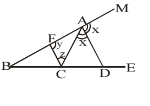Triangles - Olympiad Level MCQ, Class 10 Mathematics - Question 18

The diagonal BD of a quadrilateral ABCD bisects ∠B and ∠D, then: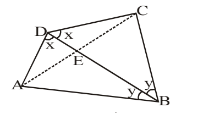Triangles - Olympiad Level MCQ, Class 10 Mathematics - Question 19

Two right triangles ABC and DBC are drawn on the same hypotenuse BC on the same side of BC. If AC and

DB intersects at P, then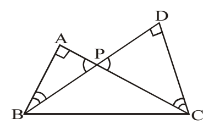Triangles - Olympiad Level MCQ, Class 10 Mathematics - Question 20

In figure, ABC is a right triangle, right angled at B. AD and CE are the two medians drawn from A and C

respectively. If AC = 5 cm and AD =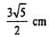, find the length of CE: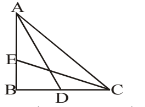Detailed Solution for Triangles - Olympiad Level MCQ, Class 10 Mathematics - Question 20

Given: A triangle ABC right angled at B. AD and CE and medians drawn from vertices A and C respectively.
To find: CE = ?
In ∆ABD, AD2 = AB2 + BD2
[Using Pythagoras theorem]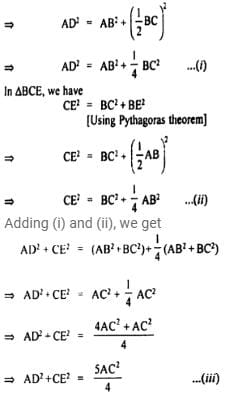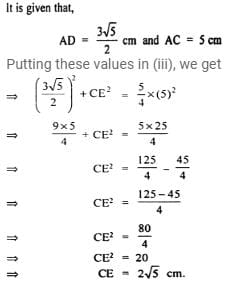Triangles - Olympiad Level MCQ, Class 10 Mathematics - Question 21

In a ΔABC, AB = 10 cm, BC = 12 cm and AC = 14 cm. Find the length of median AD. If G is the centroid,

find length of GA :

Triangles - Olympiad Level MCQ, Class 10 Mathematics - Question 22

The three sides of a triangles are given. Which one of the following is not a right triangle?

Triangles - Olympiad Level MCQ, Class 10 Mathematics - Question 23

In the figure AD is the external bisector of ∠EAC, intersects BC produced to D. If AB = 12 cm, AC = 8 cm

and BC = 4 cm, find CD.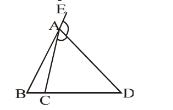Triangles - Olympiad Level MCQ, Class 10 Mathematics - Question 24

In ΔABC, AB+ AC2 = 2500 cm2 and median AD = 25 cm, find BC.

Triangles - Olympiad Level MCQ, Class 10 Mathematics - Question 25

In the given figure, AB = BC and ∠BAC = 15°, AB = 10 cm. Find the area of ΔABC.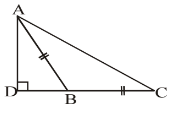## Olympiad Preparation for Class 10

39 tests
 Use Code STAYHOME200 and get INR 200 additional OFF Use Coupon Code